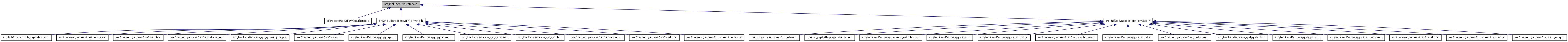# rbtree.h File Reference

This graph shows which files directly or indirectly include this file:Go to the source code of this file.

struct  RBNode

## Typedefs

typedef struct RBNode RBNode
typedef struct RBTree RBTree
typedef enum RBOrderControl RBOrderControl
typedef int(* rb_comparator )(const RBNode *a, const RBNode *b, void *arg)
typedef void(* rb_combiner )(RBNode *existing, const RBNode *newdata, void *arg)
typedef RBNode *(* rb_allocfunc )(void *arg)
typedef void(* rb_freefunc )(RBNode *x, void *arg)

## Enumerations

enum  RBOrderControl { LeftRightWalk, RightLeftWalk, DirectWalk, InvertedWalk }

## Functions

RBTreerb_create (Size node_size, rb_comparator comparator, rb_combiner combiner, rb_allocfunc allocfunc, rb_freefunc freefunc, void *arg)
RBNoderb_find (RBTree *rb, const RBNode *data)
RBNoderb_leftmost (RBTree *rb)
RBNoderb_insert (RBTree *rb, const RBNode *data, bool *isNew)
void rb_delete (RBTree *rb, RBNode *node)
void rb_begin_iterate (RBTree *rb, RBOrderControl ctrl)
RBNoderb_iterate (RBTree *rb)

## Typedef Documentation

 typedef RBNode*(* rb_allocfunc)(void *arg)

Definition at line 47 of file rbtree.h.

 typedef void(* rb_combiner)(RBNode *existing, const RBNode *newdata, void *arg)

Definition at line 46 of file rbtree.h.

 typedef int(* rb_comparator)(const RBNode *a, const RBNode *b, void *arg)

Definition at line 45 of file rbtree.h.

 typedef void(* rb_freefunc)(RBNode *x, void *arg)

Definition at line 48 of file rbtree.h.

 typedef struct RBNode RBNode
 typedef enum RBOrderControl RBOrderControl
 typedef struct RBTree RBTree

Definition at line 33 of file rbtree.h.

## Enumeration Type Documentation

 enum RBOrderControl
Enumerator:
 LeftRightWalk RightLeftWalk DirectWalk InvertedWalk

Definition at line 36 of file rbtree.h.

```{
LeftRightWalk,              /* inorder: left child, node, right child */
RightLeftWalk,              /* reverse inorder: right, node, left */
DirectWalk,                 /* preorder: node, left child, right child */
InvertedWalk                /* postorder: left child, right child, node */
} RBOrderControl;
```

## Function Documentation

 void rb_begin_iterate ( RBTree * rb, RBOrderControl ctrl )

Definition at line 838 of file rbtree.c.

Referenced by ginBeginBAScan().

```{
rb->cur = rb->root;
if (rb->cur != RBNIL)
rb->cur->iteratorState = InitialState;

switch (ctrl)
{
case LeftRightWalk:     /* visit left, then self, then right */
rb->iterate = rb_left_right_iterator;
break;
case RightLeftWalk:     /* visit right, then self, then left */
rb->iterate = rb_right_left_iterator;
break;
case DirectWalk:        /* visit self, then left, then right */
rb->iterate = rb_direct_iterator;
break;
case InvertedWalk:      /* visit left, then right, then self */
rb->iterate = rb_inverted_iterator;
break;
default:
elog(ERROR, "unrecognized rbtree iteration order: %d", ctrl);
}
}
```

 RBTree* rb_create ( Size node_size, rb_comparator comparator, rb_combiner combiner, rb_allocfunc allocfunc, rb_freefunc freefunc, void * arg )

Definition at line 114 of file rbtree.c.

Referenced by ginInitBA(), and gistrescan().

```{
RBTree     *tree = (RBTree *) palloc(sizeof(RBTree));

Assert(node_size > sizeof(RBNode));

tree->root = RBNIL;
tree->cur = RBNIL;
tree->iterate = NULL;
tree->node_size = node_size;
tree->comparator = comparator;
tree->combiner = combiner;
tree->allocfunc = allocfunc;
tree->freefunc = freefunc;

tree->arg = arg;

return tree;
}
```

 void rb_delete ( RBTree * rb, RBNode * node )

Definition at line 647 of file rbtree.c.

References rb_delete_node().

Referenced by getNextGISTSearchItem().

```{
rb_delete_node(rb, node);
}
```

 RBNode* rb_find ( RBTree * rb, const RBNode * data )

Definition at line 159 of file rbtree.c.

References RBTree::arg, cmp(), RBTree::comparator, RBNode::left, RBNIL, RBNode::right, and RBTree::root.

```{
RBNode     *node = rb->root;

while (node != RBNIL)
{
int         cmp = rb->comparator(data, node, rb->arg);

if (cmp == 0)
return node;
else if (cmp < 0)
node = node->left;
else
node = node->right;
}

return NULL;
}
```

 RBNode* rb_insert ( RBTree * rb, const RBNode * data, bool * isNew )

Definition at line 405 of file rbtree.c.

Referenced by ginInsertBAEntry(), and gistScanPage().

```{
RBNode     *current,
*parent,
*x;
int         cmp;

/* find where node belongs */
current = rb->root;
parent = NULL;
cmp = 0;                    /* just to prevent compiler warning */

while (current != RBNIL)
{
cmp = rb->comparator(data, current, rb->arg);
if (cmp == 0)
{
/*
* Found node with given key.  Apply combiner.
*/
rb->combiner(current, data, rb->arg);
*isNew = false;
return current;
}
parent = current;
current = (cmp < 0) ? current->left : current->right;
}

/*
* Value is not present, so create a new node containing data.
*/
*isNew = true;

x = rb->allocfunc (rb->arg);

x->iteratorState = InitialState;
x->color = RBRED;

x->left = RBNIL;
x->right = RBNIL;
x->parent = parent;
rb_copy_data(rb, x, data);

/* insert node in tree */
if (parent)
{
if (cmp < 0)
parent->left = x;
else
parent->right = x;
}
else
{
rb->root = x;
}

rb_insert_fixup(rb, x);

return x;
}
```

 RBNode* rb_iterate ( RBTree * rb )

Definition at line 867 of file rbtree.c.

References RBTree::cur, RBTree::iterate, and RBNIL.

Referenced by ginGetBAEntry().

```{
if (rb->cur == RBNIL)
return NULL;

return rb->iterate(rb);
}
```

 RBNode* rb_leftmost ( RBTree * rb )

Definition at line 187 of file rbtree.c.

References RBNode::left, RBNIL, and RBTree::root.

Referenced by getNextGISTSearchItem().

```{
RBNode     *node = rb->root;
RBNode     *leftmost = rb->root;

while (node != RBNIL)
{
leftmost = node;
node = node->left;
}

if (leftmost != RBNIL)
return leftmost;

return NULL;
}
```